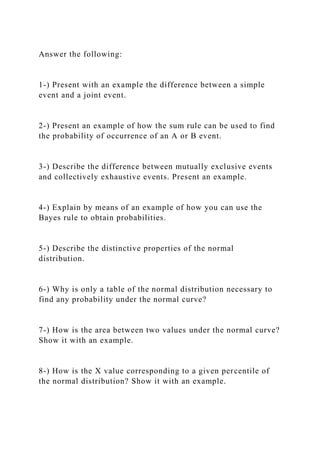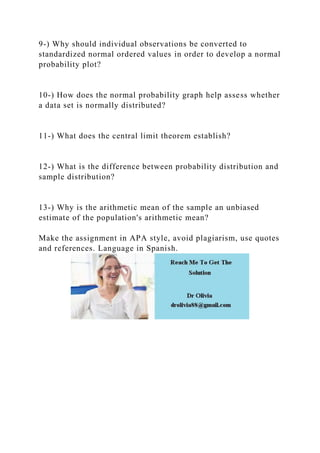Diese Präsentation wurde erfolgreich gemeldet.

# Answer the following1-) Present with an example the differenc.docx

Anzeige
Anzeige
Anzeige
Anzeige
Anzeige
Anzeige
Anzeige
Anzeige
Anzeige
Anzeige
Anzeige×

1 von 2 Anzeige

# Answer the following1-) Present with an example the differenc.docx

1-) Present with an example the difference between a simple event and a joint event.

2-) Present an example of how the sum rule can be used to find the probability of occurrence of an A or B event.

3-) Describe the difference between mutually exclusive events and collectively exhaustive events. Present an example.

4-) Explain by means of an example of how you can use the Bayes rule to obtain probabilities.

5-) Describe the distinctive properties of the normal distribution.

6-) Why is only a table of the normal distribution necessary to find any probability under the normal curve?

7-) How is the area between two values ​​under the normal curve? Show it with an example.

8-) How is the X value corresponding to a given percentile of the normal distribution? Show it with an example.

9-) Why should individual observations be converted to standardized normal ordered values ​​in order to develop a normal probability plot?

10-) How does the normal probability graph help assess whether a data set is normally distributed?

11-) What does the central limit theorem establish?

12-) What is the difference between probability distribution and sample distribution?

13-) Why is the arithmetic mean of the sample an unbiased estimate of the population's arithmetic mean?

Make the assignment in APA style, avoid plagiarism, use quotes and references. Language in Spanish.
.

1-) Present with an example the difference between a simple event and a joint event.

2-) Present an example of how the sum rule can be used to find the probability of occurrence of an A or B event.

3-) Describe the difference between mutually exclusive events and collectively exhaustive events. Present an example.

4-) Explain by means of an example of how you can use the Bayes rule to obtain probabilities.

5-) Describe the distinctive properties of the normal distribution.

6-) Why is only a table of the normal distribution necessary to find any probability under the normal curve?

7-) How is the area between two values ​​under the normal curve? Show it with an example.

8-) How is the X value corresponding to a given percentile of the normal distribution? Show it with an example.

9-) Why should individual observations be converted to standardized normal ordered values ​​in order to develop a normal probability plot?

10-) How does the normal probability graph help assess whether a data set is normally distributed?

11-) What does the central limit theorem establish?

12-) What is the difference between probability distribution and sample distribution?

13-) Why is the arithmetic mean of the sample an unbiased estimate of the population's arithmetic mean?

Make the assignment in APA style, avoid plagiarism, use quotes and references. Language in Spanish.
.

Anzeige
Anzeige

### Answer the following1-) Present with an example the differenc.docx

1. 1. Answer the following: 1-) Present with an example the difference between a simple event and a joint event. 2-) Present an example of how the sum rule can be used to find the probability of occurrence of an A or B event. 3-) Describe the difference between mutually exclusive events and collectively exhaustive events. Present an example. 4-) Explain by means of an example of how you can use the Bayes rule to obtain probabilities. 5-) Describe the distinctive properties of the normal distribution. 6-) Why is only a table of the normal distribution necessary to find any probability under the normal curve? 7-) How is the area between two values under the normal curve? Show it with an example. 8-) How is the X value corresponding to a given percentile of the normal distribution? Show it with an example.
2. 2. 9-) Why should individual observations be converted to standardized normal ordered values in order to develop a normal probability plot? 10-) How does the normal probability graph help assess whether a data set is normally distributed? 11-) What does the central limit theorem establish? 12-) What is the difference between probability distribution and sample distribution? 13-) Why is the arithmetic mean of the sample an unbiased estimate of the population's arithmetic mean? Make the assignment in APA style, avoid plagiarism, use quotes and references. Language in Spanish.